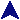Insertion SortProlog Examples```% The relation ordered_insert(X, IntoThis, GetThis) is satisfied when
% GetThis is equal to IntoThis with X added immediately before the first
% item which is greater than or equal to X.
ordered_insert(X, [], [X]).
ordered_insert(X, [Y|T], [X,Y|T]) :- X =< Y.
ordered_insert(X, [Y|T], [Y|Z]) :- X > Y, ordered_insert(X, T, Z).

% Perform sorting by insertion sort.
sorted([], []).
sorted([X|Y], Z) :- sorted(Y, SY), ordered_insert(X, SY, Z).
```